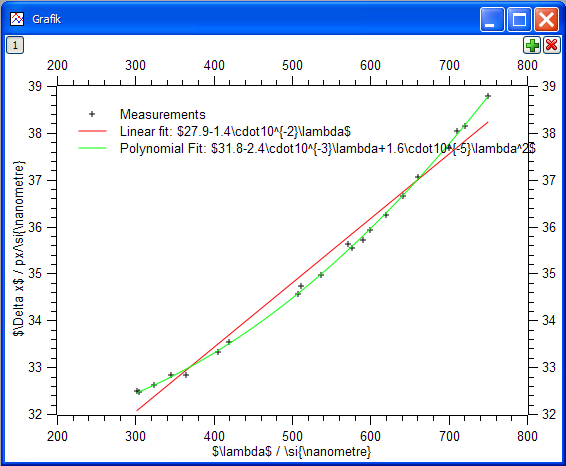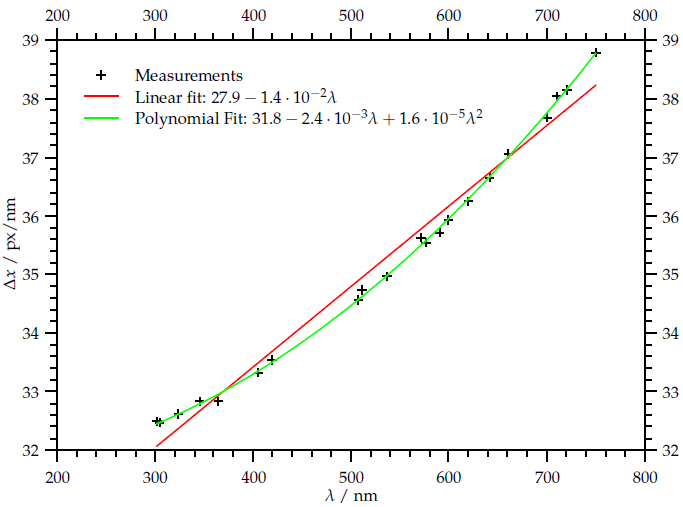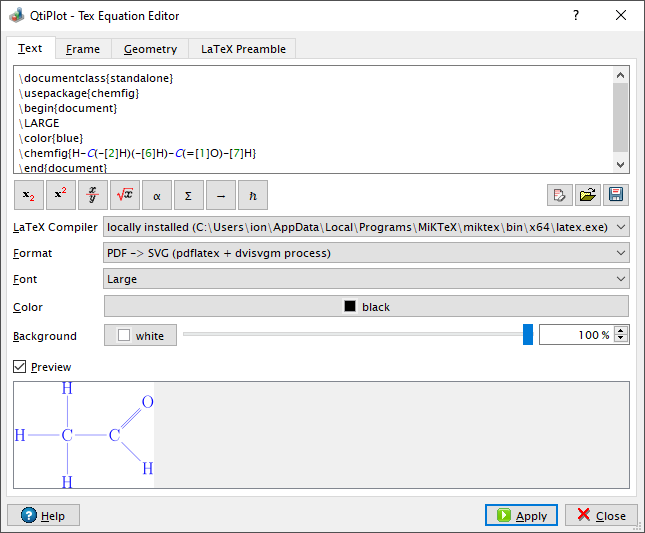# QtiPlot - Data Analysis and Scientific Plotting

 QtiPlot can be easily integrated with LaTeX, the high-quality typesetting system which is the de facto standard for the communication and publication of scientific documents: all worksheets can be exported as .tex tables and all plots can be exported as .tex figures using the TikZ/Pgf graphic systems. This makes straightforward the integration of text labels with complex mathematical expressions into the plots, like in the example bellow, showing a 2D plot with LaTeX-math mode text labels and the result image after LaTeX compilation:QtiPlot also provides a powerful equation editor making possible the compilation of complete LaTeX documents and not just of equation markup, so that you can fully customize the image output in terms of font size, text and background color: